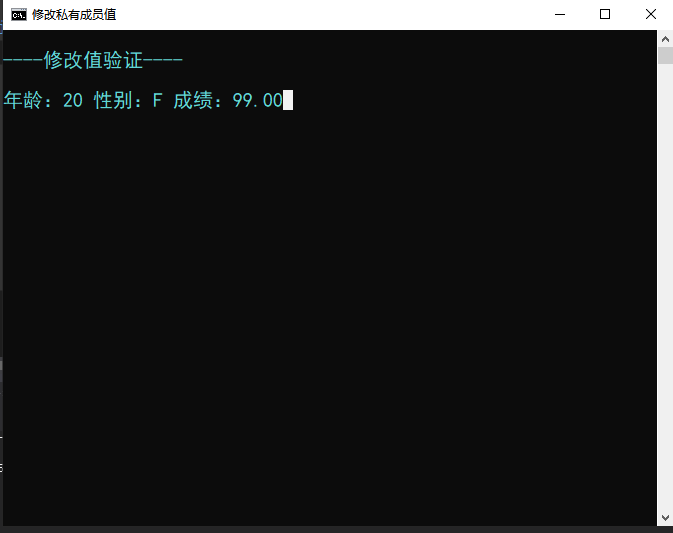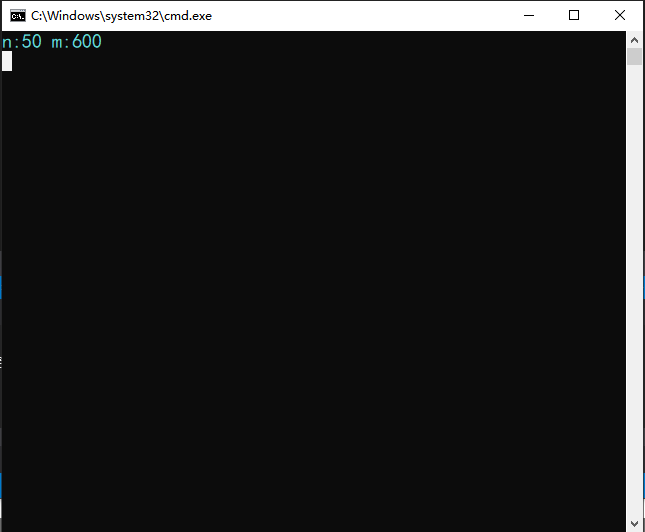## 指针解封装,你必须知道的“初级简单解法”

c语言之所以被认为强大，以及其自由性，很大部分体现在其灵活的指针运用上。

``````#include<iostream>
using namespace std;

//定义结构体
struct Student{
int age;  //年龄
char ssex;  //性别
double score;  //得分
};

int main()
{
Student stu; //创建对象
stu.age = 18;  //对象调用赋值
stu.ssex = 'F'; //对象调用赋值
stu.score = 90.00; //对象调用赋值
while (1);
}
``````

``````#include<iostream>
#include<Windows.h>
using namespace std;

struct Student{
private:
int age;  //年龄
char ssex;  //性别
double score;  //分数
public:
void show()  //定义公有成员函数，显示私有成员函数值
{
cout << endl;
cout << "----修改值验证----";
cout << endl << endl;
cout << "年龄：" << age << ' ';
cout << "性别：" << ssex << ' ' << "成绩：";
printf("%.2lf", score);
/*附：cout跟printf是不同的，cout输出float或double，
是会将小数点后非有效数字的0去掉的，而printf里面以%f输出规定是以6位小数输出，
不管是不是有效数字，这里用printf是为表面double类型的score确实被修改了*/
}

};

int main()
{
SetConsoleTitle("修改私有成员值");  //修改窗口名，头文件Windows.h
Student stu;
int *p;
p = (int*)&stu;  //p指向stu.age内存首地址  (int*)为强制类型转换
*p = 20; //修改stu.age值

p++;  //p地址偏移，指向stu.ssex
*(char*)p = 'F';  //修改stu.ssex值

p++;  //p地址继续偏移，指向stu.score
*(double*)p = 99.00;  //修改stu.score值

stu.show(); //调用公有成员函数验证私有成员age,ssex,score值确实被修改了
while (1);
}
````````````#include<iostream>
using namespace std;

int main()
{

int n = 50;
int m = 60;
int*p = &n;
p-=3;
*p = 600;
cout << "n:" << n << " " << "m:" << m;
while (1);
}

``````